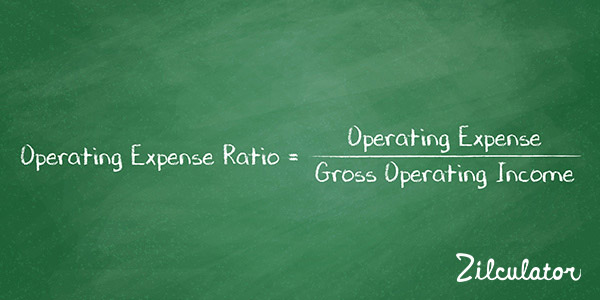# Operating Expense Ratio

## Formula & DefinitionOperating expense ratio is the ratio of individual operating expenses or of total operating expenses to the gross operating income (GOI).

Recall that the GOI is the revenue after vacancy and credit loss. Think of GOI not as the amount that you ought to collect, but rather the amount that you really collect. In that context, operating expense ratios tell you how the money you spend to operate the building relates to the money you receive.

You can use a property's expense ratios to decide if the operation of a particular investment opportunity appears typical, based on your knowledge of other similar properties. These ratios can also alert you to possible problem areas, both in regard to how the property has been run and to how accurate are the seller's representations.

It's important to look at the individual ratios of each operating expense and not just the ratio for total expenses. The total could appear entirely plausible while some of the individual items raise red flags.

## How to Calculate Operating Expense Ratio of an Investment Property

1. Calculate total operating expenses for a given year. Remember that depreciation and capital expenditures are not considered as operating expenses.
3. Use the formula below: